# Sentiment analysis with Apache MXNet

Using deep neural networks to make sense of unstructured text.

October 3, 2017Letters (source: Pixabay)

Sentiment analysis is a common task in the data science world. A company may want to monitor mentions of its products on Twitter or Facebook in order to detect (and resolve) customer satisfaction issues proactively. But human language is rich and complex; there are myriad ways to feel positive or negative emotion about something—and for each of those feelings there are, in turn, many ways to express that feeling. Among machine learning techniques for sentiment analysis, deep learning has proven to excel at making sense of these complex inputs.

In this tutorial, we’ll classify sentiment by building a neural network using Apache MXNet. Ultimately, we’ll build up to a classifier that can take the text of a brief movie review as input and try to identify it and express a positive or negative opinion about the movie. We will start with a simple dense model and then build a model similar to the convolutional architecture described in this paper by Yoon Kim. We will also visualize the output using t-sne, a visualization technique for high-dimensional data. Finally, we will use transfer learning to use the pre-built embedding glove in our neural network to classify sentences. Although there are many deep learning frameworks, such as TensorFlow, Keras, Torch, and Caffe, we’ll use Apache MXNet, which is gaining popularity due to its flexibility and scalability across multiple GPUs.

## Learn faster. Dig deeper. See farther.

Join the O'Reilly online learning platform. Get a free trial today and find answers on the fly, or master something new and useful.

I encourage you to download the notebook, where we’ve created and run all this code, and play with it! Adjust the hyperparameters and experiment with different approaches to neural network architecture or data preparation—and see if you can beat our accuracy.

This notebook expects you to have a basic understanding of convolution operation, neural networks, activation units, gradient descent, and NumPy.

By the end of the notebook, you will be able to:

1. Understand the complexity of sentiment analysis.
2. Understand word embedding and its use.
3. Prepare data sets for training the neural network.
4. Implement custom neural network architecture for classifying sentiments using various different models.
5. Visualize the result and understand our model using t-sne.
6. Use a prebuilt embedding like glove to train on data with constraints (small data set or small sentences).

## Sentiment analysis

Sentiment analysis is a complex task; understanding if a sentence expresses a positive or negative opinion is very difficult. Take a sentence like this: “The movie was unintelligent, gross, and vulgar—but I loved it.” Although the sentence contains a lot of negative words (unintelligent, gross, vulgar), the sentiment expressed is positive (“but I loved it”). Another sentence that can’t be understood simply on the basis of individual words might be: “The movie doesn’t care about cleverness, wit, or any other kind of intelligence.” Although the sentence contains a lot of positive words (cleverness, wit, intelligence), it expresses a negative review.

Some sentences are sarcastic, and/or they rely on context for their meaning (“Taken 3 makes Taken 2 a masterpiece.”). We can understand this sentence expresses a negative review because we understand the context (Taken 2 was not a great movie).

Sentiment analysis is a very difficult task due to the context, and its accuracy mostly depends on the data set that’s processed. A sentiment analyzer can perform very well on one data set and poorly on another. The machine learning developer should be aware and check if their model captures the variation in the data in order to avoid embarrassing failures.

## Encoding the data set

The futher we move from tabular data, the trickier it becomes to encode the data for processing. Compared to text, even encoding an image into numbers is a fairly straightforward process. Each pixel can only take values from 0-255 in RGB color space, and these values are in a two-dimensional array. Resizing of an image doesn’t affect the content of the image, so we can relatively easily (building on the work of image processing experts) standardize our inputs into comparable arrays.

Encoding natural language (words) into numbers, however, is not straightforward. A language can have huge vocabulary and sentences formed using those words can be of varying lengths. Sometimes, resizing sentences can change their meaning completely.

Let’s consider a toy example that helps us understand the process of encoding natural languages.
Consider a set of words, {I, love, cake, hate, pizza } that forms our entire vocabulary.
Two sentences are formed out of this vocabulary.
“I love cake”
“I hate pizza”

How can we encode theses sentences as numbers? One way is to represent the vocabulary as an encoded matrix, using one-hot encoding: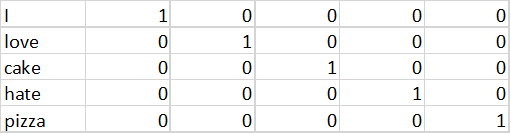In this representation, if there are N words in the vocabulary, we need a matrix of size N×N. This matrix is called a vocabulary matrix. The vocabulary matrix forms the look-up table for the word.

Now, let’s try to encode discourse. The sentence “I hate pizza” will become the following matrix: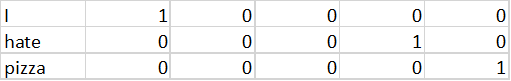If the word is present in the sentence, then the corresponding row of the vocabulary matrix is copied. This is the same operation that is performed by the embedding layer of the neural net in MXNet (or any other deep learning framework).
The “embedding layer” just performs the look-up operations and should not be confused with word-embedding (which we’ll get to shortly).

Can we do better than one-hot encoding the vocabulary? Is there a better way to represent the words? Word embedding solves this problem. Instead of discretizing the words, it provides a continuous representation of words. A word embedding matrix can look like the matrix shown below.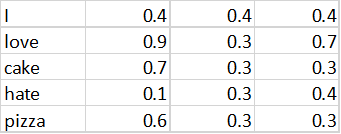Instead of representing the word as an N×N matrix, we represent the words with an N × 3 matrix, where “3” is the embedding size. So, each word can be represented as a 3-dimensional vector, instead of an N-dimensional vector.

Word embedding not only reduces the size of the representation of the vocabulary matrix, but tries to encode the semantic relationship between words. For example, “pizza” and “cake” have nearly similar word embedding vectors in this vocabulary since both refer to types of food. “Love” and “hate” have the same magnitude in 2nd dimension since they convey feelings but entirely different magnitude in 1st dimension (0.9 and 0.1, respectively) since they convey opposite sentiments.

Where do these vectors come from? These word embeddings can be learned by your deep neural network automatically during sentiment classification. The embedding vectors of particular words can be treated as weights that need to be learned by the deep neural network. These embedding techniques can also be used on images and other data; they’re commonly referred to as autoencoder networks. Basically, autoecoder tries to represent the given input in lower-dimension space with the least possible information loss. A detailed explanation can be found here.

## Convolution on sentences.

Once the sentence has been encoded into a matrix using an embedding layer, we can perform the 1D convolution on the encoded matrix. It is very similar to 2D convolution performed on 2D images.The size of the convolution filter depends on n-grams we would like to use.The convolution operation in the edges assumes the input is padded with zeros.
This convolution operation will help you learn based on the n-grams from the sequence.

Next, we will look into how sentiment classification is performed using MXNet, using a data set of actual movie review sentences.

If you’re working in the AWS Cloud, you can save yourself the installation management by using an Amazon Machine Image, pre-configured for deep learning. This will enable you to skip steps 1-5 below.

Note that if you are using a Conda environment, remember to install pip inside conda by typing conda install pip after you activate an environment. This step will save you a lot of problems down the road.

Here’s how to get set up:

1. First, get Anaconda, a package manager. It will help you to install dependent Python libraries with ease.
2. Next, install scikit learn, a general-purpose scientific computing library. We’ll use this to pre-process our data. You can install it with conda install scikit-learn.
3. Then grab the Jupyter Notebook, with conda install jupyter notebook.
4. And then, get MXNet, an open source deep learning library.

The next three steps enable visualization of the word-embedding. They’re not mandatory, but I highly recommend visualizing the results.

1. Next, we need cython, required for bhtnse
2. We also need bhtsne, a c++, python implementation of tnse. Don’t use scikit-learn’s tnse implementation, as it crashes the python kernel.
3. Finally, we need matplotlib for plots and visualization.

Here are the commands you need to type inside the anaconda environment (after its activation ):

1. conda install pip
2. pip install opencv-python
3. conda install scikit-learn
4. conda install jupyter notebook
5. pip install mxnet
6. conda install -c anaconda cython
7. pip install bhtsne
8. conda install -c anaconda matplotlib

## The data set

In order to learn about any deep neural network, we need data. For this notebook, we’ll use a movie review data set from Stanford. You can download the data set here

The data set consists of 25,000 samples in a training set and 25,000 samples in test set, with 12,500 positive and 12,500 negative sentiments in each set. For the sake of speed and simplicity, we will only be using the training set, which will be randomly split into train, validation, and test sets. As discussed earlier, sentiment analysis is a complex task, and its accuracy depends on the data set. Your model should be tested on a variety of data before deploying to production.

Here’s the code for loading the data. We assign a label of 1 for positive sentiment and 0 for negative sentiment. We won’t be one-hot encoding the labels since we will use a softmaxoutput layer in MXnet to perform classification.

In another framework, one-hot encoding the labels might be necessary.

import os
def read_files(foldername):
import os
sentiments = []
filenames = os.listdir(os.curdir+ "/"+foldername)
for file in filenames:
with open(foldername+"/"+file,"r", encoding="utf8") as pos_file:
data=pos_file.read().replace('\n', '')
sentiments.append(data)
return sentiments

#contains positive movie review    
foldername = "easy/pos"
postive_sentiment = read_files(foldername)

#contains negative movie review
foldername = "easy/neg"
negative_sentiment = read_files(foldername)

positive_labels = [1 for _ in postive_sentiment]
negative_labels = [0 for _ in negative_sentiment]


## Preparing the data set and encoding

We’ll need to start with standard data-preparation tasks. We’ll clean the data to remove URLs, special characters, etc. While you can also use the nltk library to preprocess the text, in this case we’ll use a custom function to clean the data. Once the data is cleaned, we need to form the vocabulary (all the unique words available in the data set).

Next, we need to identify the most common words in the review. This prevents relatively rare words like director name, actor name to influence the outcome of a classifier. We also map each word (sorted by descending order based on the frequency of occurrence) to a unique number (idx) which is stored in a dictionary called word_dict. We also perform the inverse mapping from the idx to the word. We use a vocabulary of 5,000 words—i.e., we’re only treating the most common 5,000 words as significant. The vocabulary size, sentence length, and embedding dimensions are also parameters, and can be experimented with while building a neural network. We use a vocabulary size of 5000, sentence length of 500 words, and embedding dimensions of size 50.

#some string preprocessing
def clean_str(string):
"""
    Tokenization/string cleaning for all datasets except for SST.
    Original taken from https://github.com/yoonkim/CNN_sentence/blob/master/process_data.py
    """
string = re.sub(r"[^A-Za-z0-9(),!?\'\]", " ", string)
string = re.sub(r"\'s", " \'s", string)
string = re.sub(r"\'ve", " \'ve", string)
string = re.sub(r"n\'t", " n\'t", string)
string = re.sub(r"\'re", " \'re", string)
string = re.sub(r"\'d", " \'d", string)
string = re.sub(r"\'ll", " \'ll", string)
string = re.sub(r",", " ", string)
string = re.sub(r"!", " ! ", string)
string = re.sub(r"$$", " ", string) string = re.sub(r"$$", " ", string)
string = re.sub(r"\?", " \? ", string)
string = re.sub(r"\s{2,}", " ", string)
string = re.sub(r",", " ", string)
string = re.sub(r"'", " ", string)
string = re.sub(r"$", " ", string) string = re.sub(r"$", " ", string)
return string.strip().lower()

#Creating a dict of words and their count in the entire dataset{word:count}
word_counter = Counter()
def create_count(sentiments):
idx = 0
for line in sentiments:
for word in (clean_str(line)).split():
if word not in word_counter.keys():
word_counter[word] = 1
else:
word_counter[word] += 1

#Assigns a unique a number for each word (sorted by descending order based on the frequency of occurrence)
#and returns a word_dict
def create_word_index():
idx = 0
word_dict = {}
for word in word_counter.most_common():
word_dict[word] = idx
idx+=1
return word_dict

#inverse mapping
idx2word = {v: k for k, v in word_dict.items()}


Next, we actually encode the sentences into numbers using the word_dict. The following code performs the operations.

#Creates encoded sentences.
#Assigns the unique id from wordict to the words in the sentences
def encoded_sentences(input_file,word_dict):
output_string = []
for line in input_file:
output_line = []
for word in (clean_str(line)).split():
if word in word_dict:
output_line.append(word_dict[word])
output_string.append(output_line)
return output_string

def decode_sentences(input_file,word_dict):
output_string = []
for line in input_file:
output_line = ''
for idx in line:
output_line += idx2word[idx] + ' '
output_string.append(output_line)
return output_string


Next, we pad or truncate the sentences to a length of 500 words. We can choose different lengths based on the sentence statistics.

def pad_sequences(sentences,maxlen=500,value=0):
"""
    Pads all sentences to the same length. The length is defined by the longest sentence.
    Returns padded sentences.
    """
padded_sentences = []
for sen in sentences:
new_sentence = []
if(len(sen) > maxlen):
new_sentence = sen[:maxlen]
padded_sentences.append(new_sentence)
else:
num_padding = maxlen - len(sen)
new_sentence = np.append(sen,[value] * num_padding)
padded_sentences.append(new_sentence)
return padded_sentences


Next, split the data into train, validation, and test sets as shown below:

#train+validation, test split
X_train_val, X_test, y_train_val, y_test_set = train_test_split(t_data, all_labels, test_size=0.3, random_state=42)

#train, validation split of data
X_train, X_val, y_train, y_validation = train_test_split(X_train_val, y_train_val, test_size=0.3, random_state=42)


## Building the deepnet

Now that we have prepared our data set, let’s actually code the neural network. Building neural networks is rather like a science—it involves a lot of experimentation. We can either choose to experiment or use a neural network that was used by researchers to solve a similar problem. We will start out with a simple dense network and then move on to complex neural network architecture.

The neural network code is concise and simple, thanks to MXNet’s symbolic API:

#A simple dense model
input_x_1 = mx.sym.Variable('data')
embed_layer_1 = mx.sym.Embedding(data=input_x_1, input_dim=vocab_size, output_dim=embedding_dim, name='vocab_embed')
flatten_1 = mx.sym.Flatten(data=embed_layer_1)
fc1_1 = mx.sym.FullyConnected(data=flatten_1, num_hidden=500,name="fc1")
relu3_1 = mx.sym.Activation(data=fc1_1, act_type="relu" , name="relu3")
fc2_1 = mx.sym.FullyConnected(data=relu3_1, num_hidden=2,name="final_fc")
dense_1 = mx.sym.SoftmaxOutput(data=fc2_1, name='softmax')


Let’s break down the code a bit. First, it creates a data layer (input layer) that actually holds the data set while training:

data = mx.symbol.Variable('data')


The vocab_embed layer performs the look up into the embedding matrix (which will be learned in the process):

embed_layer_1 = mx.sym.Embedding(data=input_x_1, input_dim=vocab_size, output_dim=embedding_dim, name='vocab_embed')


The flatten layer flattens the embedding layer weights with dimensions of seq_len X embedding dimension into a column vector of 1 X (seq_len*embedding) which serves the input for the next dense layer.

flatten_1 = mx.sym.Flatten(data=embed_layer_1)


The fully connected layers connect every neuron(output) from flatten layer(previous layer) to current layer.

mx.sym.FullyConnected(data=flatten_1, num_hidden=500,name="fc1")


The relu3_1 layer performs non-linear activation on the input for learning complex functions.

relu3_1 = mx.sym.Activation(data=fc1_1, act_type="relu" , name="relu3")


The final dense layer (softmax) performs the classification. The SoftmaxOutput layer in MXNet performs the one-hot encoding of output then applies the softmax function.

## Training the network

We are training the network using GPUs since it’s faster—up to 91% less time to train. This increases development speed and time-to-product release, of course, but also keeps development costs down. A single pass-through of the training set is referred to as one “epoch,” and we are training the network for three epochs “num_epoch = 3”. We also periodically store the trained model in a JSON file, and measure the train and validation accuracy to see our neural network “learn.”

Here is the code:

#Create Adam optimiser
adam = mx.optimizer.create('adam')

#Checkpointing (saving the model). Make sure a  folder named models exists.
model_prefix = 'model1/chkpt'
checkpoint = mx.callback.do_checkpoint(model_prefix)

#Loading the module API. Previously MXNet used feedforward (deprecated)
model =  mx.mod.Module(
context = mx.gpu(0),     # use GPU 0 for training; if you don't have a gpu use mx.cpu()
symbol = dense_1,
data_names=['data']
)

#actually fits the model for 3 epochs.
model.fit(
train_iter,
eval_data=val_iter,
batch_end_callback = mx.callback.Speedometer(batch_size, 64),
num_epoch = 3,
eval_metric='acc',
optimizer = adam,
epoch_end_callback=checkpoint
)


## Visualization

The following code will help us to visualize the result and can provide some intuition about our model. We can obtain the weights of embedding generated by our model, and then visualize the result using tnse visualization.

Visualizing vocab-size*embedding_dim (5000*500) vector in 2-d is impossible. We need to reduce the dimensionality of the data in order to visualize it. t-sne is a popular visualization technique that can reduce the dimensionality so the vectors that are closer in N (500) dimensions are close together in lower dimension(2). It basically combines the N dimension vector into a reduced dimension with minimal loss of information. t-sne is very similar to PCA.

Below is the code for extracting the embedding weights and then visualizing them as a scatter plot:

# obtains the weights of embeding layer for visualizing
params = model.get_params()
print(params)
weights_dense_embed = model.get_params()['vocab_embed_weight'].asnumpy()
weights_dense_embed = weights_dense_embed.astype('float64')

#tnse visualization for first 500 words
size = 500
Y= tsne(weights_dense_embed[:size])
plt.figure(0)
plt.scatter(Y[:, 0], Y[:, 1])
for idx, (x, y) in enumerate(zip(Y[:, 0], Y[:, 1])):
plt.annotate(idx2word[idx], xy=(x, y), xytext=(0, 0), textcoords='offset points')


Here is the t-sne visualization of the weights of top 500 words: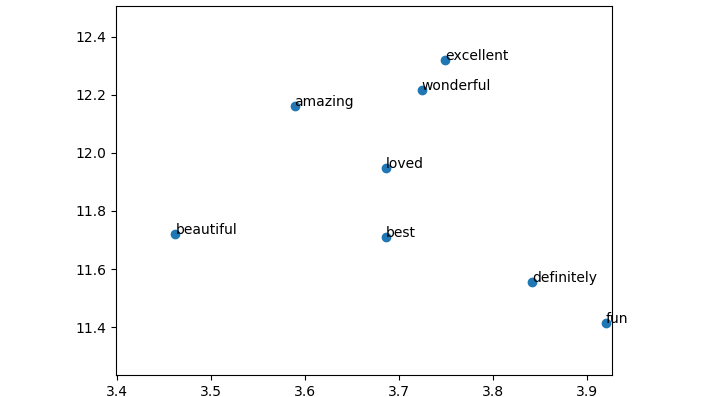The visualization shows that the model automatically learned that the words “excellent, wonderful, amazing” mean the same thing. This is quite wonderful! Next, we will write a simple predict function to use the model generated.

## Sample prediction

Let’s use this model to predict our sentences. For this, we need to load the saved model as follows:

# Load the model from the checkpoint . We are loading the 10 epoch
sym, arg_params, aux_params = mx.model.load_checkpoint(model_prefix, 3)

# Assign the loaded parameters to the module
mod = mx.mod.Module(symbol=sym, context=mx.cpu())
mod.bind(for_training=False, data_shapes=[('data', (1,500))])
mod.set_params(arg_params, aux_params)


Then encode the sentences with word_dict‘s index, and pass it to the model as a classifier.

from collections import namedtuple
Batch = namedtuple('Batch', ['data'])

#a simple predict function
def predict(sen):
# compute the predict probabilities
mod.forward(Batch([mx.nd.array(sen)]))
prob = mod.get_outputs().asnumpy()
# print the top-5
prob = np.squeeze(prob)
return prob

#our custom sentences for testing
my_sen =["the movie was awesome. Loved it"]
my_sen_encoded = encoded_sentences(my_sen,word_dict)


The output is [ 0.09290998 0.90709001], which means the classifier predicts the sentiment is positive with 0.90 probability.

This model, however, only considers single words to make predictions. We can do much better by considering the relationships between words, but in order to do that, we’ll need to build a convolutional neural network that can consider multiple consecutive words (n-grams) at a time. Next, we will look into a convolutional network for sentiment classification that can capture information in n-grams.

## Building the convolutional model.

As discussed earlier, to develop a model based on n-grams, we need a convolutional neural network. So, let’s develop one. This is very similar to the previous model but has a convolution filter of size 5.

#a model with convolution kernel of 5 (5-grams)
input_x_2 = mx.sym.Variable('data')
embed_layer_2 = mx.sym.Embedding(data=input_x_2, input_dim=vocab_size, output_dim=embedding_dim, name='vocab_embed')
conv_input_2 = mx.sym.Reshape(data=embed_layer_2, target_shape=(batch_size, 1, seq_len, embedding_dim))
conv1_2 = mx.sym.Convolution(data=conv_input_2, kernel=(5,embedding_dim), num_filter=100, name="conv1")
flatten_2 = mx.sym.Flatten(data=conv1_2)
fc2_2 = mx.sym.FullyConnected(data=flatten_2, num_hidden=2,name="final_fc")
convnet = mx.sym.SoftmaxOutput(data=fc2_2, name='softmax')


The only tricky thing is the conv_input_2 input layer. This layer reshapes the output from the embedding layer into a format that is needed by the convolutional layer. Everything else remains the same. This model can be trained using the model.fit function and various insights can be obtained.

## Building the “multiple convolution” model.

This is similar to the previous model, except that we use convolutions of different sizes (3,4,5) for developing a model based on 3-grams, 4-grams, and 5-grams, then concatenate and flatten the output. A maxpool layer is added to prevent overfitting. Below is the code in python:

# a model with convolution filters of 3, 4, 5 (3-gram,4-grams,5-grams)
input_x_3= mx.sym.Variable('data')
embed_layer_3 = mx.sym.Embedding(data=input_x_3, input_dim=vocab_size, output_dim=embedding_dim, name='vocab_embed')
conv_input_3 = mx.sym.Reshape(data=embed_layer_3, target_shape=(batch_size, 1, seq_len, embedding_dim))

# create convolution + (max) pooling layer for each filter operation
filter_list=[3, 4, 5] # the size of filters to use

num_filter=100
pooled_outputs = []
for i, filter_size in enumerate(filter_list):
convi = mx.sym.Convolution(data=conv_input_3, kernel=(filter_size, embedding_dim), num_filter=num_filter)
relui = mx.sym.Activation(data=convi, act_type='relu')
pooli = mx.sym.Pooling(data=relui, pool_type='max', kernel=(seq_len - filter_size + 1, 1), stride=(1,1))
pooled_outputs.append(pooli)

# combine all pooled outputs
total_filters = num_filter * len(filter_list)
concat = mx.sym.Concat(*pooled_outputs, dim=1)

# reshape for next layer
h_pool_3 = mx.sym.Reshape(data=concat, target_shape=(batch_size, total_filters))
fc2_3 = mx.sym.FullyConnected(data=h_pool_3, num_hidden=2,name="final_fc")
convnet_combined = mx.sym.SoftmaxOutput(data=fc2_3, name='softmax')


## Transfer learning from glove

The embedding we generated using the neural network gives us lot of insights. But to generate an embedding, we need a lot of data. What if we are restricted to a small amount of data? Transferring weights from a different pre-trained neural network can be very useful. This helps to build a model even with a small amount of data.

Here, we will use the glove embedding developed by Stanford. We will use the Wikipedia – 2014 glove embedding, which was trained on a Wikipedia corpus of six billion words. The embedding itself has 400k unique words (the vocabulary) and contains embedding with various dimensions (50,100,200,300). We will use the 50 dimension embedding for training the neural network. The word embedding dimension is also a hyper parameter and you should experiment with different values to see what gives you the best results. The following functions load the embedding vector into an embedding matrix (numPy matrix):

#loads glove word embedding
def load_glove_index(loc):
f = open(loc,encoding="utf8")
embeddings_index = {}
for line in f:
values = line.split()
word = values
coefs = np.asarray(values[1:], dtype='float32')
embeddings_index[word] = coefs
f.close()
return embeddings_index

#creates word embedding matrix
def create_emb():
embedding_matrix = np.zeros((vocab_size, embedding_dim))
for word, i in word_dict.items():
if i >= vocab_size:
continue
embedding_vector = embeddings_index.get(word)
if embedding_vector is not None:
# words not found in embedding index will be all-zeros.
embedding_matrix[i] = embedding_vector
return embedding_matrix
embeddings_index = load_glove_index("glove/" + 'glove.6B.50d.txt')
embedding_matrix = create_emb();


Let’s visualize the embedding of glove using t-sne:

#visualization of word embedding.
size = 500
Y= tsne(embedding_matrix[:size])
plt.figure(1)
plt.scatter(Y[:, 0], Y[:, 1])
for idx, (x, y) in enumerate(zip(Y[:, 0], Y[:, 1])):
plt.annotate(idx2word[idx], xy=(x, y), xytext=(0, 0), textcoords='offset points')


Here is the t-sne visualization of the first 500 words glove embedding: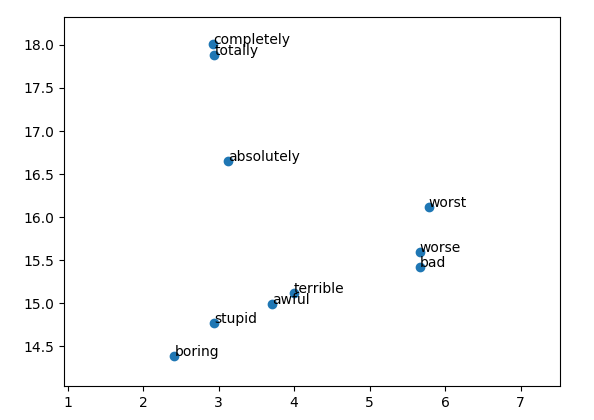As you can see, the embedding has grouped similar words (worse, worst, bad, terrible) together. Let’s use this embedding to create a neural network to classify sentences. Adding the pre-trained weights to the neural network can be little tricky. Below is the code:

#creates a model with convolution and pre-trained word embedding.
weight_matrix = mx.nd.array(embedding_matrix)
input_x_3= mx.sym.Variable('data')
the_emb_3 = mx.sym.Variable('weights') #the weight variable which will hold the pre trained embedding matrix
embed_layer_3 = mx.sym.Embedding(data=input_x_3,weight=the_emb_3, input_dim=vocab_size, output_dim=embedding_dim, name='vocab_embed')
conv_input_3 = mx.sym.Reshape(data=embed_layer_3, target_shape=(batch_size, 1, seq_len, embedding_dim))
conv1_3 = mx.sym.Convolution(data=conv_input_3, kernel=(5,embedding_dim), num_filter=100, name="conv1")
flatten_3 = mx.sym.Flatten(data=conv1_3)
fc2_3 = mx.sym.FullyConnected(data=flatten_3, num_hidden=2,name="final_fc")
convnet_word2vec = mx.sym.SoftmaxOutput(data=fc2_3, name='softmax')


Here, we first convert the glove embedding_matrix into an mx.nd.array. Then we create a symbol variable named weights and assign it to a variable the_emb_3. This variable is passed as a parameter to the embed_layer_3 in mx.sym.Embedding.

The next step is to train the neural network by passing the weight_matrix as the default weights for the embed_layer_3. Also, we need to freeze the weights of the embedding layer so that, during back propagation, the weights of embed_matrix are not updated. Remember to pass fixed_param_names =['weights'] in the Module API to freeze the weights of embedding layer. Also, pass the arg_params={'weights': weight_matrix} while fitting to use the glove embedding weights. Below is the python code:

adam = mx.optimizer.create('adam')

#Checkpointing (saving the model). Make sure there is folder named model4 exist
model_prefix_3 = 'model4/chkpt'
checkpoint = mx.callback.do_checkpoint(model_prefix_3)

model_3 =  mx.mod.Module(
context = mx.gpu(0),     # use GPU 0 for training; if you don't have a gpu use mx.cpu()
symbol = convnet_word2vec,
fixed_param_names =['weights'] # makes the weights variable non trainable. Back propagration will not update #this variable
)

#fits the model for 5 epochs.
model_3.fit(
train_iter,
eval_data=val_iter,
batch_end_callback = mx.callback.Speedometer(batch_size, 64),
num_epoch = 5,
eval_metric='acc',
epoch_end_callback=checkpoint,
arg_params={'weights': weight_matrix}, #loads the pretrained glove embedding to weights variable
allow_missing= True
`

You probably noticed that using glove word-embedding (pre-trained word embedding) for this particular data set does not provide a better model. Experimenting with different dimensions of word-embedding and using a higher capacity model (more neurons) may improve performance. Download the notebook and see if you can make it work better! You might also consider experimenting with recursive neural networks like LTSM or GRU to improve performance.

## Conclusion

In this tutorial, we performed sentiment classification on a movie review data set. We developed models of varying complexity using MXNet and understood the important concept of embedding and a way to visualize the weights learned by our model. In the end, we also performed transfer learning using glove embedding.

In our next tutorial, we will learn how deep learning can be used to generate new images, sound, etc. These types of models are called generative models.

Post topics: Artificial Intelligence
Post tags: Apache MXNet Tutorials
Share: